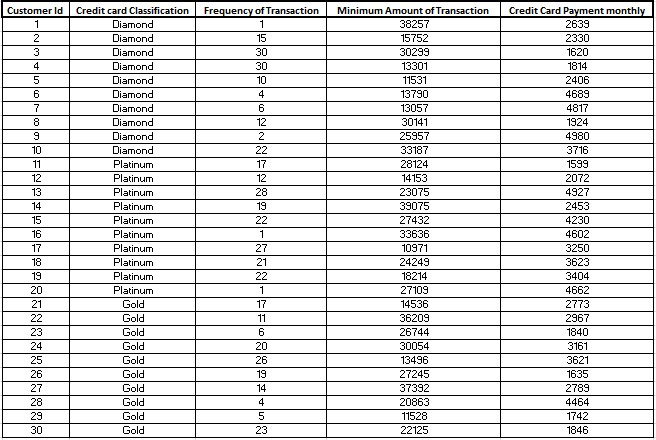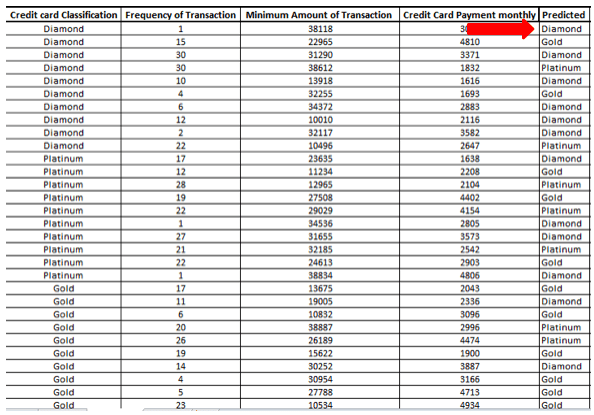# How to apply linear discriminant analysis?

Linear discriminant model is a multivariate model. It is used for modelling the differences in groups. In this model, a categorical variable can be predicted through a continuous or binary dependent variable.  The linear discriminant analysis allows researchers to separate two or more classes, objects and categories based on the characteristics of other variables. It is a classification technique like logistic regression. However, the main difference between discriminant analysis and logistic regression is that instead of dichotomous variables, discriminant analysis involves variables with more than two classifications.

For example, discriminant analysis helps determine whether students will go to college, trade school or discontinue education.

Therefore, it works on the possible patterns in student’s attributes that can decide students’ categorization. This categorization is done on the basis of patterns of selected attributes.

For example, in case of student’s score, family income or student’s participation in co-curricular activities are the attributes.

Here discriminant analysis will treat these variables, i.e. student’s score, family income or student’s participation as independent variables to predict a student’s classification. Hence, in this case, the dependent variable has three more categories. Therefore, logistic regression is not compatible in such cases.

## How linear discriminant analysis works

Linear discriminant analysis creates an equation which minimizes the possibility of wrongly classifying cases into their respective groups or categories.  It includes a linear equation of the following form:

```D = a1*X1 + a2*X2 + ……… + ai*Xi + b,

where:
D= discriminant function

X-= responses for the variable (attributes)

“a” = discriminant coefficient

B = constant, and

“i”= number of discriminant variables.```

Similar to linear regression, the discriminant analysis also minimizes errors. It also iteratively minimizes the possibility of misclassification of variables. Therefore, choose the best set of variables (attributes) and accurate weight for each variable to minimize the possibility of misclassification.

## Assumptions of discriminant analysis

Discriminant analysis works on some strong assumptions. These assumptions mark its difference from logistic regression, which are:

1. There must be two or more groups or categories.
2. There must be at least two respondents (observational units, like students in the above case).
3. The number of discriminating variables in the model must be less than the total number of respondents minus 2.
4. Discriminating variables are measured at the interval or ratio scale level. Dummy variables also work well.
5. No discriminating variable may be a linear combination of the other discriminating variables.
6. The covariance matrices must be approximately equal for each group, except for cases using special formulas.
7. Each group derives from a population with normal distribution on the discriminating variables. Group sizes should not be too different, otherwise, the units will tend to have overprediction of membership in the largest group.

## Example of linear discriminant analysis

This section explains the application of this test using hypothetical data. The case involves a dataset containing categorization of credit card holders as ‘Diamond’, ‘Platinum’ and ‘Gold’ based on a frequency of credit card transactions, minimum amount of transactions and credit card payment. Therefore, the aim is to apply this test in classifying the cardholders into these three categories.Case dataset for linear discriminant analysis

The first step is to test the assumptions of discriminant analysis which are:

• Normality in data.
• Variables should be exclusive and independent (no perfect correlation among variables).
• Homogenous variance.

SPSS software was used for conducting the discriminant analysis. Results are as follows:

### Eigenvalues

Function Eigenvalue/td> % of Variance Cumulative % Canonical Correlation
1 .091a 66.6 66.6 .289
2 .046a 33.4 100.0 .209
a. First 2 canonical discriminant functions were used in the analysis.
Eigenvalues from the discriminant analysis in SPSS

Eigenvalues shows the discriminating ability of the function. These values are the matrix product from the inverse function of the “within groups sum of squares”. Similarly, the canonical correlation values are the correlation between the grouping of the dependent variable and the predictor variables.

### Wilks’ Lambda

Test of Function(s)

Wilks’ Lambda

Chi-square

df

Sig.

1 through 2

.876

3.435

6

.753

2

.956

1.165

2

.558

Wilks’ lambda values from the discriminant analysis in SPSS

Similarly, the Wilks’ lambda is another statistical output from the discriminant analysis. In this case, the Wilks’ lambda is calculated by using the following equation.

`Wilks’ lambda = [1- (0.289)2]* [1-(0.209)2]`

The tables below explain the results. The first table shows the classification results. Here notice that the classification of ‘Diamond’ shows 50% prediction accuracy by test attributes (variables). Consequently, the classification of ‘Platinum’ and ‘Gold’ shows 30% and 20% accuracy in prediction by test variables.

 Classification Results Classification Predicted Group Membership Total Diamond Platinum Gold Original Count Diamond 6 2 2 10 Platinum 4 3 3 10 Gold 2 2 6 10 % Diamond 60.0 20.0 20.0 100.0 Platinum 40.0 30.0 30.0 100.0 Gold 20.0 20.0 60.0 100.0 Cross-validated Count Diamond 5 3 2 10 Platinum 4 3 3 10 Gold 4 4 2 10 % Diamond 50.0 30.0 20.0 100.0 Platinum 40.0 30.0 30.0 100.0 Gold 40.0 40.0 20.0 100.0 a. 50.0% of original grouped cases correctly classified. b. Cross-validation is done only for those cases in the analysis. In cross-validation, each case is classified by the functions derived from all cases other than that case. c. 33.3% of cross-validated grouped cases correctly classified. Prediction from the discriminant analysis in SPSS.

Furthermore, the table below represents the predicted results of the discriminant analysis of the above case.Prediction from the discriminant analysis in SPSS

## Application of discriminant analysis

Application of discriminant analysis is similar to that of logistic regression. However, it requires additional conditions fulfilment suggested by assumptions and presence of more than two categories in variables. Also, discriminant analysis is applicable in a small sample size, unlike logistics regression. A few instances where discriminant analysis is applicable are; evaluation of product/ service quality. Furthermore, banks also use it for promotional strategies.

Lastly, software that supports linear discriminant analysis are R, SAS, MATLAB, STATA and SPSS.

### Priya Chetty

Partner at Project Guru
Priya is a master in business administration with majors in marketing and finance. She is fluent with data modelling, time series analysis, various regression models, forecasting and interpretation of the data. She has assisted data scientists, corporates, scholars in the field of finance, banking, economics and marketing.

### Related articles

• How to apply logistic regression in a case? Machine learning involves solutions to predict scenarios based on past data. Logistic regression offers probability functions based on inputs and their corresponding output.
• Understanding random operating curves or ROC analysis Receiver Operating Curve (ROC) is an extension of such classifications. Performance of binary classifier system in the case of ROC analysis can be tested.
• How to perform nonlinear regression? Regression analysis is a statistical tool to study the relationship between variables. These variables are the outcome variable and one or more exposure variables. In other words, regression analysis is an equation which predicts a response from the value of a certain predictor.
• How to perform and apply Monte Carlo simulation? Monte Carlo simulation is an extension of statistical analysis where simulated data is produced. This method uses repeated sampling techniques to generate simulated data.
• How to conduct generalized least squares test? In statistics, Generalized Least Squares (GLS) is one of the most popular methods for estimating unknown coefficients of a linear regression model when the independent variable is correlating with the residuals.We are looking for candidates who have completed their master's degree or Ph.D. Click here to know more about our vacancies.